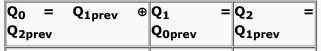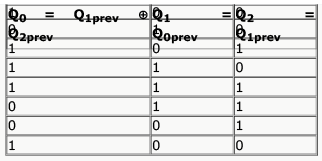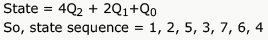Courses

# Synchronous And Asynchronous Circuits Practice Quiz - 1

## 10 Questions MCQ Test RRB JE for Computer Science Engineering | Synchronous And Asynchronous Circuits Practice Quiz - 1

Description
This mock test of Synchronous And Asynchronous Circuits Practice Quiz - 1 for Computer Science Engineering (CSE) helps you for every Computer Science Engineering (CSE) entrance exam. This contains 10 Multiple Choice Questions for Computer Science Engineering (CSE) Synchronous And Asynchronous Circuits Practice Quiz - 1 (mcq) to study with solutions a complete question bank. The solved questions answers in this Synchronous And Asynchronous Circuits Practice Quiz - 1 quiz give you a good mix of easy questions and tough questions. Computer Science Engineering (CSE) students definitely take this Synchronous And Asynchronous Circuits Practice Quiz - 1 exercise for a better result in the exam. You can find other Synchronous And Asynchronous Circuits Practice Quiz - 1 extra questions, long questions & short questions for Computer Science Engineering (CSE) on EduRev as well by searching above.
QUESTION: 1

###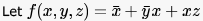be a switching function. Which one of the following is valid?

Solution:

f(x,y,z) = x' + y'x + xz
An implicant of a function is a product term that is included in the function. so x', y'x and xz ,all are implicants of given function.
A prime implicant of a function is an implicant that is not included in any other implicant of the function.
option a)   y'x is not a prime implicant as it is included in xz [ xy'z+ xyz]
option d) y is not a prime implicant as it include in both x' and xz.
a product term in which all the variables appear is called a minterm of the function

QUESTION: 2

### Which are the essential prime implicants of the following Boolean function?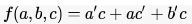Solution:

using K map f = ac' + a'c

QUESTION: 3

### A main memory unit with a capacity of 4 megabytes is built using 1M x 1-bit DRAM chips. Each DRAM chip has 1K rows of cells with 1K cells in each row. The time taken for a single refresh operation is 100 nanoseconds. The time required to perform one refresh operation on all the cells in the memory unit is

Solution:

There are 4*8 = 32 DRAM chips to get 4MB from 1M x 1-bit chips. Now, all chips can be refreshed in parallel so do all cells in a row. So, the total time for refresh will be number of rows times the refresh time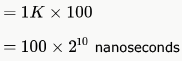*Answer can only contain numeric values
QUESTION: 4

A ROM is used to store the Truth table for a binary multiple unit that will multiply two 4-bit numbers. The size of the ROM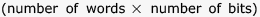that is required to accommodate the Truth table is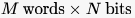. Write the values of M and N.

Solution:

A is 4 bit binary no A4A3A2A1
B is 4 bit binary no B4B3B2B1
M is result of multiplication M8M7M6M5M4M3M2M1     [check biggest no 1111 x 1111 =11100001]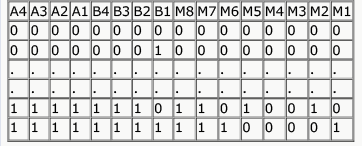So 4 bit of A 4 bit of B
input will consist of 8 bit need address 00000000 to 11111111 = 28 address
output will be of 8 bits
so memory will be of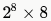M = 256 , N = 8

QUESTION: 5

A ROM is used to store the table for multiplication of two 8-bit unsigned integers. The size of ROM required is

Solution:

When we multiply two 8 bit numbers result can go up to 16 bits. So, we need 16 bits for each of the multiplication result.
Number of results possible = 28 ⨯ 28 = 216 = 64 K as we need to store all possible results of multiplying two 8 bit numbers. So, 64 K ⨯ 16 is the answer.

QUESTION: 6

What is the minimum size of ROM required to store the complete truth table of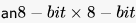multiplier?

Solution:

multiplying 2 8 bit digits will give result in maximum 16 bits
total number of multiplications possible = 28 x 28
hence space required = 64K x 16 bits

QUESTION: 7

The amount of ROM needed to implement a 4 - bit  multiplier is

Solution:

A ROM cannot be written. So, to implement a 4 bit multiplier we must store all the possible combinations of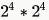input bits and  output bits giving a total of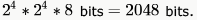. So, (D) is the answer.

QUESTION: 8

Which of the following input sequences for a cross-coupled R-S flip-flop realized with two NAND gates may lead to an oscillation?

Solution:

For R-S flip flop with NAND gates (inputs are active low) 11-no change 00-indeterminate..............so option A may make the system oscillate as "00" is the final input. In option D, after "00" flipflop output may oscillate but after "11", it will be stabilized.

QUESTION: 9

The below figure shows four D-type flip-flops connnected as a shift register using an XOR gate. The initial state and three subsequent states for three clock pulses are also given.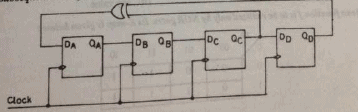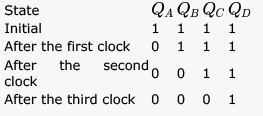The state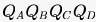after the fourth clock pulse is

Solution: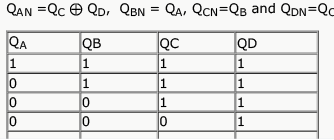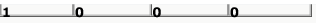QUESTION: 10

Consider the circuit given below with initial state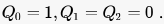. The state of the circuit is given by the value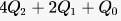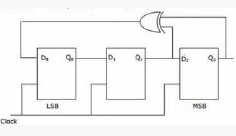Which one of the following is correct state sequence of the circuit?

Solution: